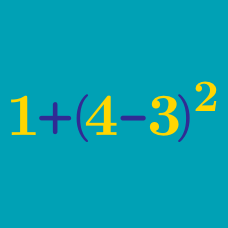Everyday Math

# Representation on the Real Line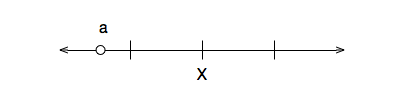In the above real line, if $$X = 3$$, which of the following is a possible value for $$a?$$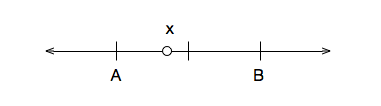In the above real line, if $$A = 2$$ and $$B = 4$$, which of the following is a possible value for $$x$$?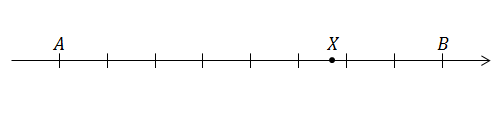If $$X=-10.28$$ on the real line above, and the distance between each pair of marks is 1, what is the value of $$A+B?$$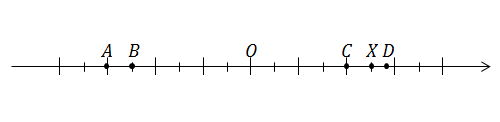Which of the following represents the number that has the same absolute value as $$X?$$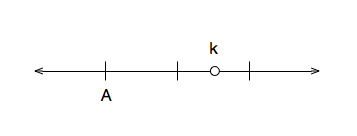In the above number line, if $$k = 4.4$$, which of the following is a possible value for $$A$$?

Note: in the image above, each tick mark represents one unit.

×